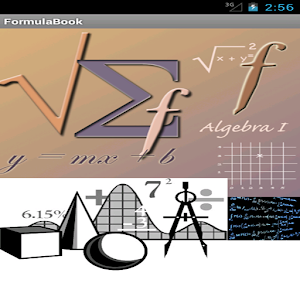# Formula 5001Free - Lumos Educational App Store1
Price -
\$

#### DESCRIPTION:

Math Formula is part of Formula 5001 is a simple application that helps you access a vast collection of math formulas, with thousands of mathematical equations. Here you will get all basic, intermediate and advance formula for children, 10th standard, 12th standard and graduation level. All kinds of Mathematics Formula 1. Basic Math Formula All basic Mathematic formulla from arithmetic, speed, loss & profite 2.Calculus Formula All basic and advanced level formulla from calculus. Limits Derivatives Integrals Laplace Transforms 3. Algebra Formula Basic Properties and Fact

#### OVERVIEW:

Formula 5001Free is a free educational mobile app By Keshima Technologies Pvt Ltd .It helps students in grades 8 practice the following standards .

This page not only allows students and teachers download Formula 5001Free but also find engaging Sample Questions, Videos, Pins, Worksheets, Books related to the following topics.

8

Developer: Keshima Technologies Pvt Ltd

Software Version: 1

Category: Education

### RELATED APPS:EdSearch WebSearch